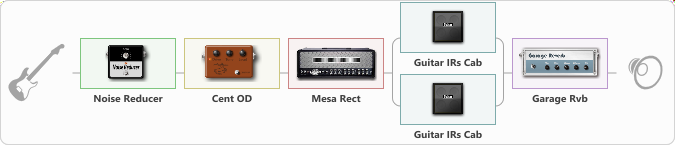Discussion in 'ToneLib-GFX presets' started by Sarcoramphus, Oct 15, 2020.

Metal Sound for Low tuning 6ers and 7 Strings

Effects chain:Effect: "Noise Reducer" (Dynamics / Filter), active - "yes"
{
"Sens" = 81
"Mode" = Soft
}

Effect: "Cent OD" (Overdrive / Distortion), active - "yes"
{
"Drive" = 40
"Tone" = 70
"Level" = 74
}

Effect: "Mesa Rect" (Amp simulators), active - "yes"
{
"Gain" = 55
"Bass" = 50
"Middle" = 50
"Treble" = 55
"Presence" = 55
"Master" = 60
"Level (dB)" = 0
}

Effect: "Splitter" (Dynamics / Filter), active - "yes"
{
"A-Bypass" = Off
"A-Pan" = 0
"A-Level" = 55
"B-Bypass" = Off
"B-Pan" = 0
"B-Level" = 55

'A' branch:
{

Effect: "Guitar IRs Cab" (Cabinets), active - "yes"
{
"Model" = Engl Pro (4x12")
"Mic Position" = Edge
"Mic Distance" = Near
"Low Cut (Hz)" = 80
"Hi Cut (kHz)" = 15.0
"Mix" = 100
"Level (dB)" = 5
}
}
'B' branch:
{

Effect: "Guitar IRs Cab" (Cabinets), active - "yes"
{
"Model" = Engl Pro (4x12")
"Mic Position" = Middle
"Mic Distance" = Near
"Low Cut (Hz)" = 80
"Hi Cut (kHz)" = 15.0
"Mix" = 100
"Level (dB)" = 5
}
}
}

Effect: "Garage Rvb" (Reverberation), active - "yes"
{
"Time" = 5.2
"PreDelay" = 46
"LoDamp" = 0
"HiDamp" = 30
"Mix" = 10
}

Note: You will need to download and install the ToneLib-GFX software to use the preset.